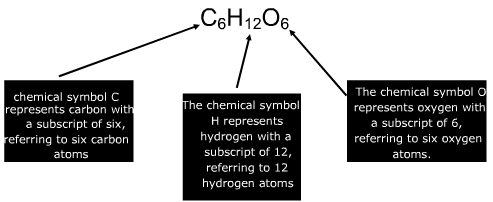# Molecular Formula

Molecular Formula

A molecular formula describes the number and kinds of atoms represented in a molecule of a substance. A molecule refers to two or more atoms bonded together by sharing electrons. Typically they consist of two or more non metals. Here is an example of a glucose molecule.Molecular Formula Questions:

1. Describe the number and types of atoms in a molecule of carbon tetrachloride – CCl4.

Answer:

The symbol C represents the element carbon. It does not have a subscript so only one carbon atom is present.

The symbol Cl represents the element chlorine. It has a subscript of four, representing four atoms of chlorine.

2. Describe the number and types of atoms in a molecule of PF5.

Answer:

The symbol P represents the element phosphorous. It does not have a subscript so only one phosphorous atom is present.

The symbol F represents the element fluorine. It has a subscript of five, representing five atoms of fluorine. The name of this molecule is phosphorous penta fluoride.

 Related Links: Lewis Structures & Molecular Shapes Quiz Polarity II: Molecular Shape Quiz Empirical & Molecular Formulas Quiz Intermolecular Forces Quiz Amedeo Avogadro Facts Compounds Examples